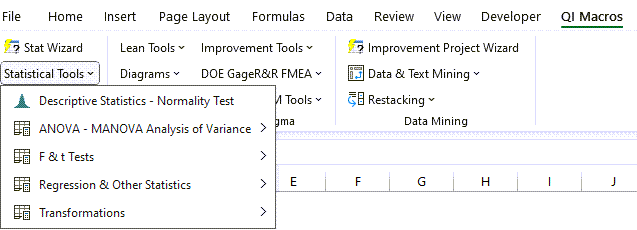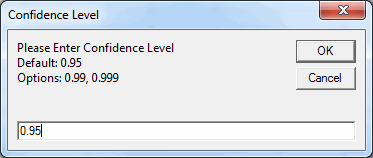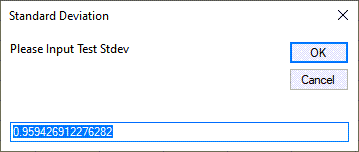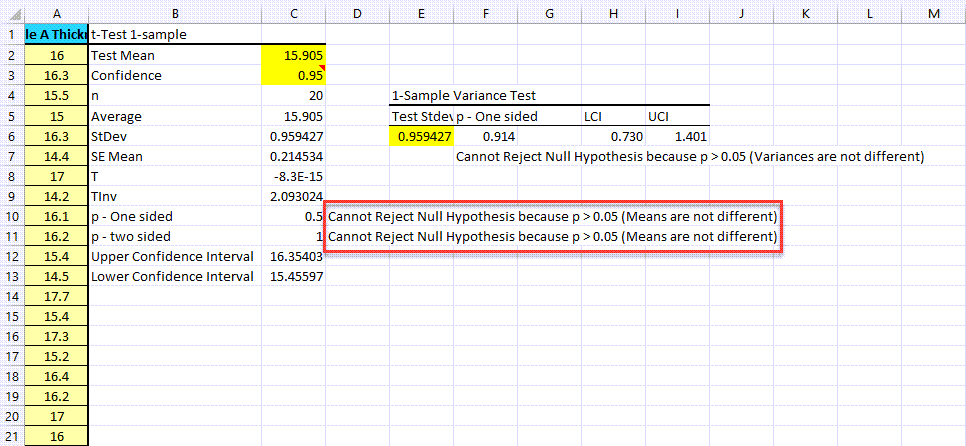# Need to Run a One-Sample Variance Test in Excel?

## QI Macros can do it for you and interpret the results!

#### Run a one-sample variance test using QI Macros

2. Click on QI Macros menu > Statistical Tools > F & t Tests > Variance Test One-Sample
3. QI Macros will do the math and interpret the results for you.

### Example of One-Sample Variance Test Using QI Macros Add-in for Excel

1. Click and drag over your data to select it in Excel:
2.3. Click on the QI Macros Menu > Statistical Tools > F & t Tests, and then select "Variance-Test: one-sample":
4.5. QI Macros will prompt for a significance level (default = 0.95):
6.7. You will then be prompted to input the Test Standard Deviation (QI Macros will provide the auto-calculated value):
8.9. QI Macros will perform the Variance Test: one-sample calculations and interprets the results for you:
10.NOTE: We do not provide Bonett method results - only Chi-Square method results.

### What's Cool about the QI Macros One Sample Hypothesized Variance Test?

Interprets the Results for You: QI Macros compares the p value to alpha and tells you what that means. In the above example, QI Macros tells you "Cannot Reject the Null Hypothesis/Accept the Null Hypothesis", because p > 0.05 (Means are not different/Means are the same).

Interactive Results: QI Macros lets you change the significance level to see what impact those changes have on your results.